﻿ Performance Evaluation of Multivariate Adaptive Regression Splines (MARS) and Multiple Linear Regression (MLR) for Forward Conversion of Geodetic Coordinates (ϕ, λ, h) to Cartesian Coordinates (X, Y, Z)Publications are Open
Access in this journal
Article Versions
Export Article
• Normal Style
• MLA Style
• APA Style
• Chicago Style
Research Article
Open Access Peer-reviewed

### Performance Evaluation of Multivariate Adaptive Regression Splines (MARS) and Multiple Linear Regression (MLR) for Forward Conversion of Geodetic Coordinates (ϕ, λ, h) to Cartesian Coordinates (X, Y, Z)

M.S. Peprah , I.O. Mensah, J. A. Akresi
Journal of Geosciences and Geomatics. 2017, 5(3), 109-118. DOI: 10.12691/jgg-5-3-2
Published online: May 03, 2017

### Abstract

In Ghana’s local Geodetic Reference Network, the standard forward transformation equation has played a major role in coordinate transformation between World Geodetic System 1984 (WGS84) and local geodetic datum. Thus, it is an initial step in forward conversion of geodetic coordinates (ϕ, λ, h) to Cartesian coordinates (X, Y, Z) in transformation from global to local datum and vice versa. Several studies in the recent decades have been conducted on converting Cartesian coordinates to geodetic coordinates (reverse procedure) through the utilisation of iterative, approximate, closed form, vector-based and computational intelligence algorithms. However, based on the existing literature covered pertaining to this present study, it was found that the existing knowledge do not fully adhere to the issue of evaluating alternative techniques in the case of the forward conversion. Hence, the aim of this present study was to explore the coordinate conversion performance of the Multivariate Adaptive Regression Splines (MARS) and Multiple Linear Regression (MLR). The performance of each model was assessed based on statistical indicators of Mean Square Error (MSE), Root Mean Square Error (RMSE), Mean Bias Error (MBE), Mean Absolute Error (MAE), Standard Deviation (SD), Noise to Signal Ratio (NSR), Correlation Coefficient (R), and Correlation of Determination (R2). The statistical findings revealed that the MARS and MLR offered satisfactory prediction of Cartesian coordinates. However, the MLR compared to MARS showed better stability and more accurate prediction results. From the results of this present study, the main conclusion drawn is that, MLR provides a promising alternative in the forward conversion of geodetic coordinates into Cartesian coordinates. Therefore, the capability of MLR as a powerful tool for solving majority of function approximation problems in mathematical geodesy has been demonstrated in this present study.

### 1. Introduction

Global Navigation Satellite System (GNSS) such as the Global Positioning System (GPS) in capturing locations of stationary and non-stationary objects on, above, and beneath the Earth’s surface has increased the possibility of obtaining coordinate positions with improved high accuracy and precision 1. These satellite positioning technologies provide numerous amounts of spatio-temporal datums in either curvilinear geodetic coordinates (ϕ, λ, h) or Cartesian coordinate (X, Y, Z) system. In order to solve most practical GPS navigation, geodetic, cartographical and astro-observation problems, it is important to convert geodetic coordinates (ϕ, λ, h) into Cartesian coordinates (X, Y.Z) and vice versa 1, 2, 3, 4, 5. The process of converting geodetic coordinates to Cartesian coordinates is known as the forward conversion 1.

The forward conversion is an initial step in converting the GPS position measurement to the local coordinate system 6, 7. This is because before the invention of the GPS, local geodetic datums were established based on traditional surveying techniques such as triangulation, trilateration, traversing, astronomical observation and among others 8, 9. Hence, the local geodetic datum involved data in only geodetic coordinates without the existence of Cartesian coordinates 1. This prevalent situation has been problematic over the years to utilize GPS coordinates based on the global datum of world geodetic system 1984 (WGS84) into a local geodetic system. The first approach in utilizing GPS data locally requires the determination of transformation parameters. The most widely adopted techniques in the past decades in literature for such application include the similarity models of Bursa-Wolf, Molodensky-Badekas, Veis model and three-dimensional Affine 10, 11, 12, 13, 14, 15, 16. However, the major concern here is that, before these aforementioned similarity models could be applied, there is the need to convert all the geodetic dataset of common points to Cartesian coordinates. It must be well noted here that, without such conversion, the similarity models cannot be adopted in the transformation parameter determination for coordinate transformation between the global and local datums 1.

In order to accomplish this task, the standard forward transformation equation 17, 18, 19, 20 given by Equation (1) to (3) are mainly widely used technique as the first step in the coordinate transformation procedure given as: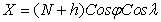(1)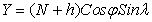(2)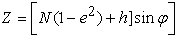(3)

Where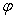,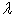and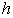are the geodetic latitude, geodetic longitude and geodetic height while X, Y, Z are the Cartesian coordinates. N in Equation (1) to (3) is the radius of curvature in the prime vertical 1 defined by Equation (4) as: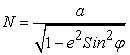(4)

Where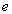is the first eccentricity expressed by Equation (5) as: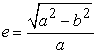(5)

Where a and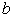are the semi-major axis and semi-minor axis of the geodetic ellipsoid 1.

Although Equation (1) to (3) are the mostly adopted technique in geodetic studies, there exist other numerical methods such as Artificial Neural Network (ANN), Multivariate Adaptive Regression Splines (MARS), Multiple Linear Regression (MLR) and many more that can serve as alternative approach. It is well acknowledged that the introduction of artificial intelligence techniques applications has revolutionized the field of mathematical geodesy in terms of its attainable accuracy and also in most instance, its dominance over the empirical methods. This assertion is well archived in existing technical papers of mathematical geodesy. For example, ANN has been applied to solve most coordinate transformation problems global and local datums 1, 9, 21, 22, 23, 24, 25, 26, 27, 28, 29, and transforming from geodetic coordinates to Cartesian coordinates 2 and many more. ANNs are been criticized for its long training process in achieving the optimal network’s topology, and it is not easy to identify the relative importance of potential input variables, and certain interpretive difficulties 30, 31.

There has been a surge of interests within the geodetic community in the recent decades in converting Cartesian coordinates into geodetic coordinates and this process is known as the reverse coordinate conversion technique. Several studies have been conducted such as iterative, approximate, closed form, vector-based algorithms 32, 33, 34, 35, 36 and artificial intelligence algorithms 1, 2. Hence, Multivariate Adaptive Regression Splines (MARS) and Multiple linear regression (MLR) were applied in this study to ascertain its ability to convert geodetic coordinates to Cartesian coordinates (forward conversion).

Multivariate Adaptive Regression Splines (MARS) is an adaptive modelling process invented by 37 used for non-linear relationships. In addition, MARS divides the predictor variables into piece-wise linear segments to describe non-linear relationships between the predictor and the dependent variable 31, 38. There is limited availability of literature of MARS in transformation of coordinates studies but many studies have successfully applied MARS for solving different problems in engineering. Some of the areas of applications include estimating energy demand 39, slope stability analysis 31, 40, 41, 42, landslide susceptibility mapping 43, water pollution prediction 44, earthquake modelling 45, studying ecological variables 46, region spatio-temporal mapping 47, modelling of the ionosphere 48, geothermal prospect 49 and so on.

Multiple Linear Regression technique (MLR) was also applied in this study as an alternative mathematical procedure to carry out the forward conversion from geodetic coordinates to Cartesian coordinates. Several studies have been carried out using MLR and Simple Linear Regression (SLR) procedures in coordinate transformation from global to local datum and vice versa 1, 50, 51, 52, 53. It is well acknowledged that, the regression techniques achievable are applicable to surveying and mapping related works 1. In addition, this present study adopted the MLR over the SLR based on the multiple input parameters utilized in Equation (1) to (3) to carry out the forward coordinate conversion. This will help maintain system consistency between MLR and Equation (1) to (3). The objective of this present study is to evaluate and compare the performance of MARS and MLR models in converting geodetic coordinates to Cartesian coordinates.

MLR have been applied in coordinate transformation but MARS have not been applied in both coordinate transformation and in coordinate conversion. The difference is that, in coordinate conversion as in the case of this present study, there is no change of datum and the geodetic ellipsoid parameters chosen for the transformation process are all based on the same geodetic datum 1. That is, the geodetic datums used in coordinate transformation, the source and target coordinate reference systems are all based on different datums and the transformation parameters are empirically determined and thus maybe subject to measurement errors 1.

The existing knowledge and publications have not fully addressed the issue of applying alternative techniques in the forward conversion of geodetic coordinates to Cartesian coordinates. In addition, upon careful review of existing studies, the authors realized that the utilization of the MARS and MLR techniques have not applied as a practical alternative technology to the existing approaches. This present study for the first time explored the utilization of the MARS and MLR in the forward conversion of geodetic coordinates to Cartesian coordinates. To achieve the aim of this present study, the MARS and MLR methods were applied. This study also highlights the comparison between MARS and MLR through the use of a set of training and test data based on the results of statistic performance indicators such as mean (M), mean square error (MSE), root mean square error (RMSE), mean bias error (MBE), mean absolute error (MAE), standard deviation (SD), noise to signal ratio (NSR), correlation of coefficient (R), and correlation of determination (R2). The finding of these two models will reveal the working efficiency of the two models for converting geodetic coordinate to Cartesian coordinates. Hence this study will serve as an added contribution to existing knowledge of MARS and MLR in mathematical geodesy.

### 2. Resources and Methods Used

The study area (Figure 1) is situated in the mining town of Tarkwa which is the administrative capital of the Tarkwa Nsuaem Municipal Assembly in the Western Region of Ghana. It is found in the Southwest of Ghana with geographical coordinates between longitudes 1° 59′ 00″ W and latitude 5° 18′ 00″ N and is 78 m above mean sea level. It is about 85 km from Takoradi, which is the regional capital, 233 km from Kumasi and about 317 km from Accra 52. The topography is generally described as remarkable series of ridges and valleys. The ridges are formed by the Banket and Tarkwa Phyllites whereas upper quartzite and Huni Sandstone are present in the valleys. Surface gradients of the ridges are generally very close to the Banket and Tarkwa Phyllites. Its environs generally lie within the mountain ranges covered by thick forest interjected by undulating terrain with few scarps. The study area has a South-western Equatorial climate with seasons influenced by the moist South-West Monsoon winds from the Atlantic Ocean and the North-East Trade Winds. The mean rainfall is approximately 1500 mm with peaks of more than 1700 mm in June and October. Between November and February, the rainfall pattern decrease to between 20 mm to 90 mm 54. The mean annual temperature is approximately 25°C with small daily temperature variations. Relative humidity varies from 61 % in January to a maximum of 80 % in August and September 52, 55.

• Figure 1. Study Area

In this present study, a total of 328 GPS geodetic coordinates (ϕ, λ, h) collected by field measurement in Tarkwa, Ghana, situated in West Africa, were used in the MARS and MLR model formulation. It is well acknowledged that one of the contributing factors affecting the estimation accuracy of models is related to the quality of datasets used in model-building and selection of appropriate inputs parameters 56, 57. Therefore, to ensure that the obtained geodetic coordinate (ϕ, λ, h) data from the GPS receivers are reliable and accurate, several factors such as checking of overhead obstruction, obstruction, observation period, observation period, observation principles and techniques as suggested by many researchers 58. In addition, all potential issues relating to GPS survey work were also considered. The next factor considered in this present study was the identification of the input parameters for the MARS and MLR training. It must be noted here that the input parameters act as control variables with an influence on the desired output of the models. Hence, the input variable which are the (independent variables) should represent the condition for which training of the two models are done 59. Consequently, the 328 GPS points measurement was first transformed into Cartesian coordinates (X, Y, Z) using Equation (1) to (3). The GPS world geodetic system 1984 (WGS84) semi-major axis,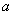and semi-minor axis,parameter values of 6378137.0 m and 6378299.99899 m were implemented in Equation (1) to (3). It is important to note that, in order for the MARS and MLR models to have consistency with Equation (1) to (3), the radius of curvature in the prime vertical (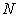) and the square of the square of the first eccentricityvalues were estimated separately. With this in mind,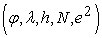was used as the input layer data while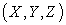was used as the output layer data. Table 1 and Table 2 are sample of the dataset that was used for the study.

2.1. Methods
2.1.1. Multivariate Adaptive Regression Splines (MARS)

The MARS model uses a nonparametric modelling approach that does not require assumptions about the form of the relationship between the independent and dependent variables 37, 60. The MARS model works by dividing the ranges of the explanatory variables into regions and by producing for each of these regions a linear regression equations 60. The breaks values between each regions are called knots, whiles the term basis functions (BFs) are used to demonstrate each distinct interval of the predictors 45, 60. BFs are functions of the following form as denoted by Equation (1):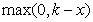(6)

Where x is an independent variable and k is a constant corresponding to a knot 60. The general formula for the MARS model is given by Equation (7) as denoted by 31: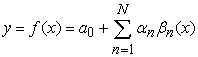(7)

Where,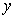is the dependent variable predicted by the function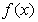,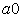is a constant, andis the number of terms, each of them formed by a coefficient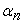and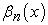is an individual basis functions or a product of two or more BFs. The MARS model was developed in two steps. In the first step, the forward algorithm, basis functions are presented to define Equation (7). Many basis functions are added in Equation (7) in order to get a better result 45, 60. The developed MARS may experience overfitting due to the large number of basis functions. In order to mitigate this problem, the second step that is the backward algorithm prevents overfitting by removing redundant basis functions from Equation (7). The MARS model adopts Generalized Cross-Validation (GCV) to delete the redundant basis functions 61. The expression of GCV is written as given by Equation (8) 62: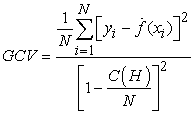(8)

Whereis the number of data and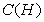is a complexity penalty that increases with the number of basis function (BFs) in the model and which is defined as denoted by Equation (9):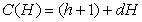(9)

Where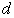is a penalty for each BFs included into the model and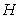is the number of basis functions in Equation (7) 37, 61.

2.1.2. Multiple Linear Regression (MLR)

The multiple linear regression (MLR) is an extensively used techniques in geoscientific studies for articulating the dependence of a response variable on several explanatory variables 1. It fits a linear combination of the components of multiple input parameters to a single output parameter defined by Equation (10) as: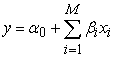(10)

Where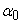is the intercept (values when all the independent variables are zero) with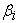values denoting the regression coefficients which were obtained in this present study using the least square technique. In Equation (10),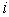is an integer varying from 1 to M, where M is the total number of observations. Since there are several variables that can be used as candidates for predictor variables in the MLR model formulation, it would be demanding having to try every possible combination of variables 1.

2.1.3. Model Performance Assessment

In order to compare the MARS and MLR models results, the residuals calculated between the desired outputs and the outputs produced by the various techniques were utilized. Hence, to make an objective assessment of the models, performance criteria indices (PCI) of mean error (ME), mean square error (MSE), root mean square error (RMSE), mean bias error (MBE), standard deviation (SD), noise to signal ratio (NSR), correlation coefficient (R), correlation of determination (R2) were adopted. Their mathematical representation is given by Equation (11) as: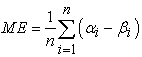(11)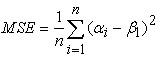(12)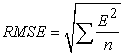(13)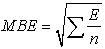(14)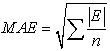(15)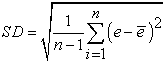(16)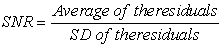(17)

With reference to Equations (11) to (15),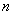is the total number of points,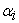andare the measured variable and estimated variable produced by the models.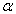represent the residuals between the measured and estimated variables and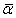is the mean value of the residuals.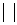in Equation (15) represents the absolute value of the residuals estimated betweenand.The correlation coefficient (R) is given by Equation (18) as: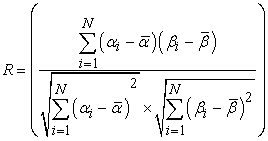(18)

Whereis the total number of test examples presented to the learning algorithms,and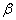are the measured and predicted plane coordinates from the various procedures, whileand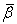are the mean of the predicted plane coordinates.in Equation (16) denotes the residual between the measured and predicted plane coordinates, and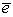is the average of the residual. The R2 was computed by Equation (19) as: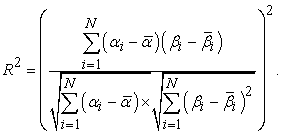(19)

### 3. Results and Discussions

The training and testing results for the two models are represented by Figure 2 to Figure 7, sample of the results is shown in Table 3 to Table 5 and their statistical analysis are tabulated in Table 6 and Table below. From the figures below, it was observed that, the performance of MLR was encouraging as compared to MARS. MLR achieved satisfactory results with high accuracy as compare to MARS which achieved good results but with less accuracy predictive performance.

• Figure 2. Training results by the two models
• Figure 3. Testing results by the two models
• Figure 4. Training results by the two models
• Figure 5. Testing results by the two models

From Figure 2 and Figure 3, it can be observed that MLR model has achieved better results in estimating the X coordinates as compared to the MARS model. 25 basis function was used in the formulation of the MARS model. The final equation that was obtained for the training and testing by the MARS model is given by Equation 20: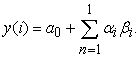(20)

The optimal equation developed for the training and testing by the MLR model is given by Equation 21: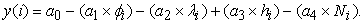(21)

The results achieved by the two models in estimating Y coordinates is represented by Figure 4 and Figure 5. Sample of the results is tabulated in Table 4 below. From the results, it was observed that, the MLR achieved better results in estimating the Y coordinates as compared to the MARS model.

The results achieved by the two models in estimating the Z coordinates is represented by Figure 6 and Figure 7. Sample of their results is tabulated in Table 5 below. From the Figure 6 and Figure 7, it can be observed that MLR model achieved higher results in estimating the Z coordinates as compare to the MARS model which achieved satisfactory results but with less accuracy.

• Figure 6. Training results obtained by the two models
• Figure 7. Testing results obtained by the two models
• Figure 8. Correlation results

The statistical performance of both models is shown in Table 6 and Table 7. From the statistical findings, it was observed that MLR achieved higher performance in terms of accuracy.

The correlation coefficient and correlation of determination which shows the relationship between the dependent variables (output) and independent variables (input) is represented by Figure 8. From the Figure 8, it was observed that, there was a higher relationship between the dependent and independent variables in estimating the Cartesian coordinates.

### 4. Conclusions

Transformation of geodetic coordinates whose reference is the World Geodetic System 1984 (WGS84) into a local system have been a common practice in mathematical geodesy for solving major problems of astronomic, geodetic, cartographic, navigation and datum related issues. This procedure is generally carried out using the standard forward transformation equation given Equation (1) to Equation (3). However, existing knowledge has shown that, little or no alternative techniques has been carried out to serve as a substitute for the standard forward transformation equation. The main contribution in this present study is to evaluate the working efficiency of MARS and MLR models as a realistic alternative technology for converting geodetic coordinates to Cartesian coordinates. The utilization of MARS and MLR for conversion of geodetic coordinates to cartesian coordinates have been presented in this study. The statistical findings revealed that the MLR and MARS offered satisfactory results in estimating Cartesian coordinates. However, MLR compared to MARS showed superior stability and more accurate results. It can be therefore be proposed that, the MLR should be used instead of the MARS within the study area in the forward conversion of geodetic coordinates to Cartesian coordinates. On the basis of the analysis achieved in this present study, it has demonstrated that geodetic longitude (λ), geodetic latitude (ϕ), geodetic height (h), radius of curvature (N) in the prime vertical and first eccentricity (e) squared, combined into MLR could produce accurate estimate of Cartesian coordinates. Therefore, this study does not only have a localized significance but will also open up more scientific discourse into the applications of MLR and MARS in mathematical geodesy within the geoscientific community.

### Acknowledgements

The authors would like to thank the anonymous reviewers for their helpful comments and also to thank all Professors across the globe for their impartation of knowledge in all fields of study. Special thanks go to the staff members of the Department of Geomatic Engineering for providing us the required instruments used for the collection of data that was used to undertake this study.

### ReferencesThis work is licensed under a Creative Commons Attribution 4.0 International License. To view a copy of this license, visit http://creativecommons.org/licenses/by/4.0/